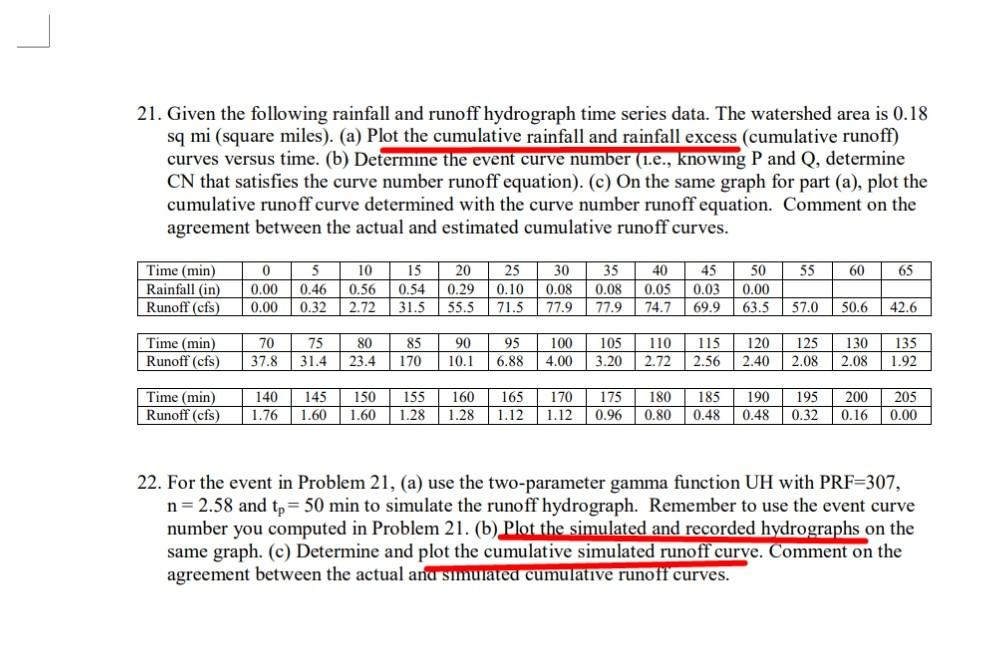# Question   solve Q21 in 60 mins i will give thumb up I need hand written solution on pages donot give typed or any previously uploaded solution 21. Given the following rainfall and runoff hydrograph time series data. The watershed area is $$0.18$$ sq mi (square miles). (a) Plot the cumulative rainfall and rainfall excess (cumulative runoff) curves versus time. (b) Determine the event curve number (1.e., knowing $$\mathrm{P}$$ and $$\mathrm{Q}$$, determine $$\mathrm{CN}$$ that satisfies the curve number runoff equation). (c) On the same graph for part (a), plot the cumulative runoff curve determined with the curve number runoff equation. Comment on the agreement between the actual and estimated cumulative runoff curves. 22. For the event in Problem 21, (a) use the two-parameter gamma function UH with PRF=307, $$\mathrm{n}=2.58$$ and $$\mathrm{t}_{\mathrm{p}}=50 \mathrm{~min}$$ to simulate the runoff hydrograph. Remember to use the event curve number you computed in Problem 21. (b) Plot the simulated and recorded hydrographs on the same graph. (c) Determine and plot the cumulative simulated runoff curve. Comment on the agreement between the actual and simulated cumulative runoff curves.RRGGY8 The Asker · Civil Engineeringsolve Q21 in 60 mins i will give thumb up
I need hand written solution on pages
donot give typed or any previously uploaded solution

Transcribed Image Text: 21. Given the following rainfall and runoff hydrograph time series data. The watershed area is $$0.18$$ sq mi (square miles). (a) Plot the cumulative rainfall and rainfall excess (cumulative runoff) curves versus time. (b) Determine the event curve number (1.e., knowing $$\mathrm{P}$$ and $$\mathrm{Q}$$, determine $$\mathrm{CN}$$ that satisfies the curve number runoff equation). (c) On the same graph for part (a), plot the cumulative runoff curve determined with the curve number runoff equation. Comment on the agreement between the actual and estimated cumulative runoff curves. 22. For the event in Problem 21, (a) use the two-parameter gamma function UH with PRF=307, $$\mathrm{n}=2.58$$ and $$\mathrm{t}_{\mathrm{p}}=50 \mathrm{~min}$$ to simulate the runoff hydrograph. Remember to use the event curve number you computed in Problem 21. (b) Plot the simulated and recorded hydrographs on the same graph. (c) Determine and plot the cumulative simulated runoff curve. Comment on the agreement between the actual and simulated cumulative runoff curves.
More
Transcribed Image Text: 21. Given the following rainfall and runoff hydrograph time series data. The watershed area is $$0.18$$ sq mi (square miles). (a) Plot the cumulative rainfall and rainfall excess (cumulative runoff) curves versus time. (b) Determine the event curve number (1.e., knowing $$\mathrm{P}$$ and $$\mathrm{Q}$$, determine $$\mathrm{CN}$$ that satisfies the curve number runoff equation). (c) On the same graph for part (a), plot the cumulative runoff curve determined with the curve number runoff equation. Comment on the agreement between the actual and estimated cumulative runoff curves. 22. For the event in Problem 21, (a) use the two-parameter gamma function UH with PRF=307, $$\mathrm{n}=2.58$$ and $$\mathrm{t}_{\mathrm{p}}=50 \mathrm{~min}$$ to simulate the runoff hydrograph. Remember to use the event curve number you computed in Problem 21. (b) Plot the simulated and recorded hydrographs on the same graph. (c) Determine and plot the cumulative simulated runoff curve. Comment on the agreement between the actual and simulated cumulative runoff curves.